## 5.2.3 Check for Mid LSF stability

26.4473GPPCodec for Enhanced Voice Services (EVS)Error concealment of lost packetsRelease 17TS

The interpolation of the mid-LSF can create unstable LSFs under packet erasure conditions. Let theth sub-frame LSFs are given by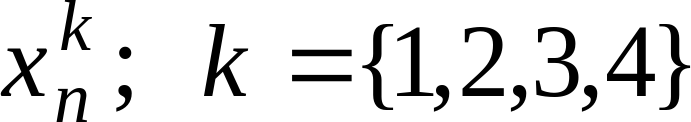. We denote the last sub-frame LSF ofth frame as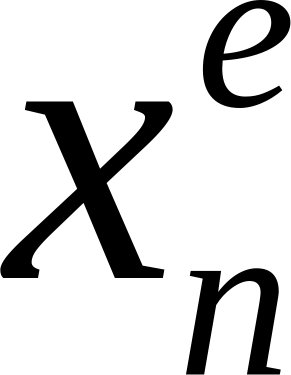where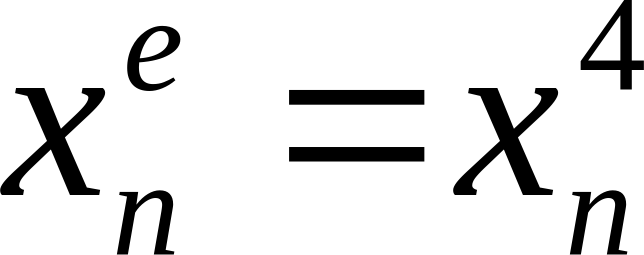. Let us denote theth LSF dimension of theth sub-frame of frame n as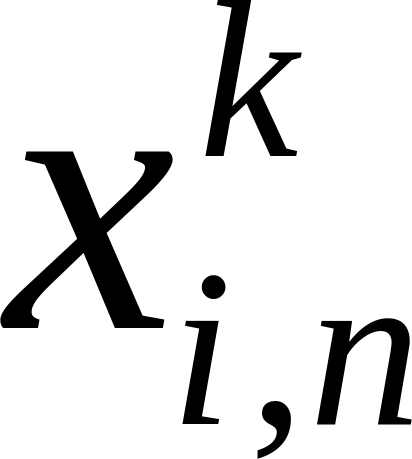where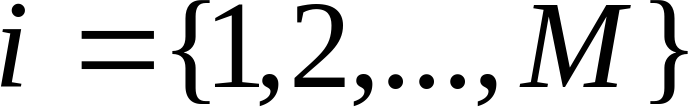.

The end-LSF quantizer quantizes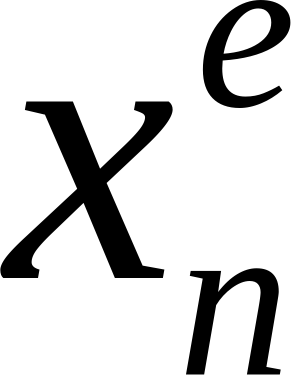. Then mid-LSF quantizer interpolates the mid-LSFs as follows.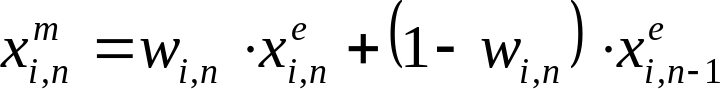(21)

where i-th dimension of the weighting vectoris given by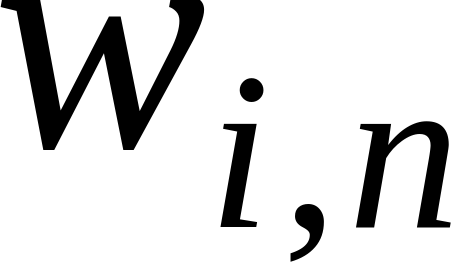. The vector elements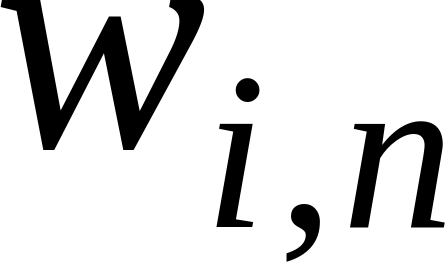are not constrained. In particular ifinterpolation generates a mid-LSF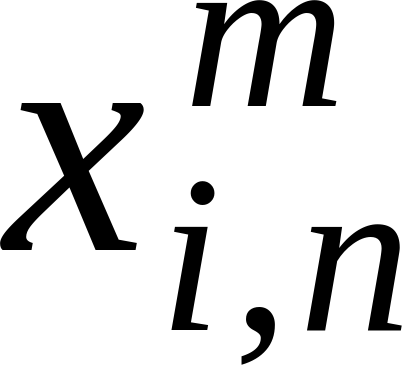between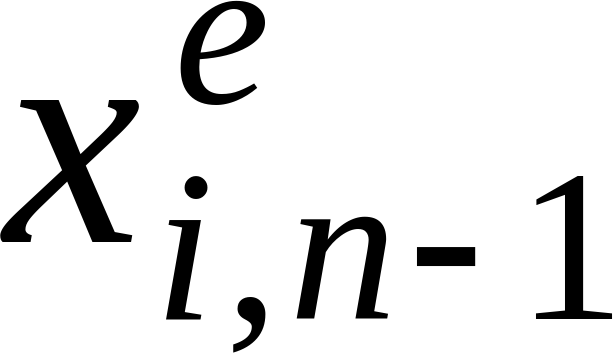and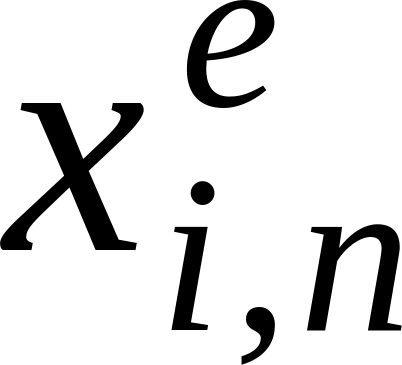. However if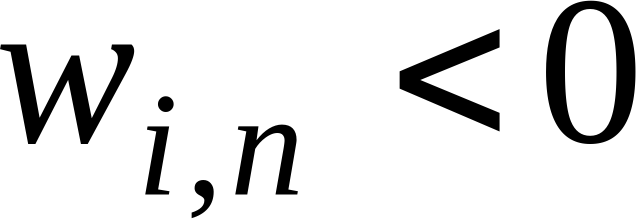or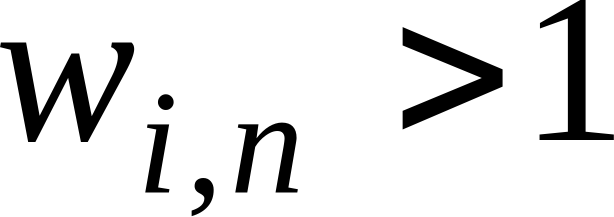interpolation might generate a mid-LSF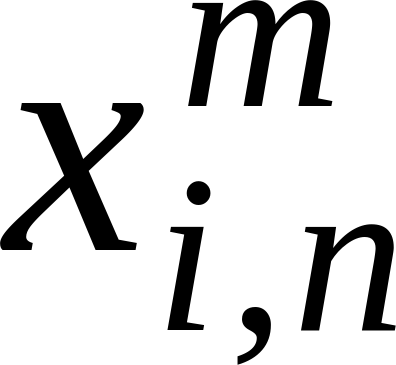outside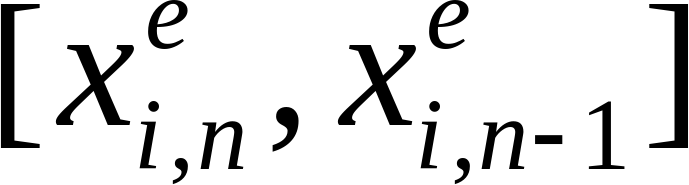. This could potentially create LSF clustering that result in an unstable LSF synthesis filter. To remedy this situation, a potential instability is detected as described below.

In the frame that follows the packet loss, the decoder checks whether the computed mid-LSFs are ordered correctly i.e.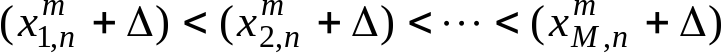. If violation of this rule is detected the LSFs are considered as potentially unstable. If potential LSF instability is detected, decoder uses a fixed weighting value(typically 0.6) for mid LSF interpolation as follows.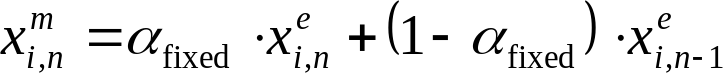(22)

The mid LSF interpolation based on equation (22) is continued until framesuch that the frameis the first frame after framethat uses safety net quantization for quantizing its end LSF.